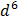# Ifis the minimum distance between the curvesand, then the value ofis

## Question ID - 153420 :- Ifis the minimum distance between the curvesand, then the value ofis

3537

(8)Since the graphs ofandare symmetrical about the lineminimum distance is the distance along the common normal to both the curves, i.e.,must be parallel to the tangent as both the curves are inverse of each otherandandNext Question :
 The least integral value ofwhereis monotonically decreasing is